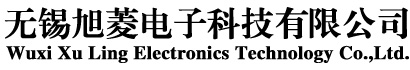JV3000变频器在注塑机上应用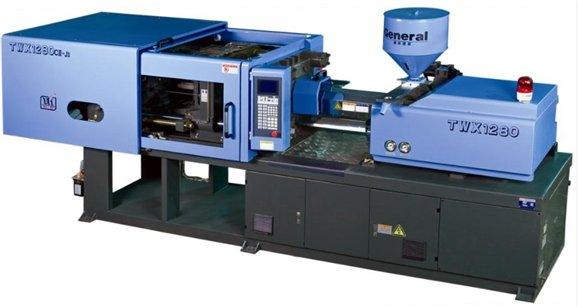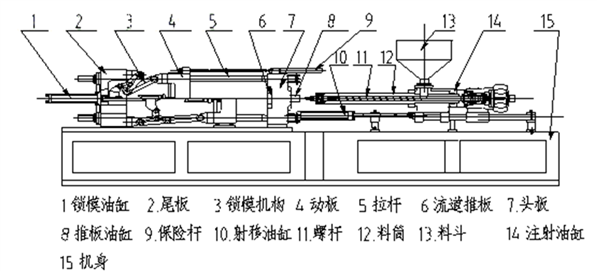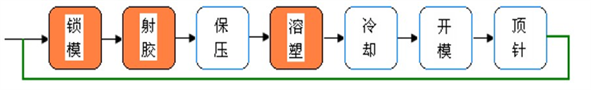. 注塑机节能原理： 除了电动式注塑机，其它都属于液压传动注塑机，液压传动系统中的动力由电机带动油泵提供。在注塑周期过程中的变化，注塑机在不同工序下需要的流量和压力不同，必须依靠流量阀和压力阀调节不同工序所须的流量和压力,由于定量泵不可调节输出功率，因此多余的能量只能在挡板，油路泄露，这部分泄露的能量就是我们要节约的能量所在.节能装置根据注塑工艺要求提供所需能量,不再有多余的能量泄露是所有注塑节能改造的最基本依据。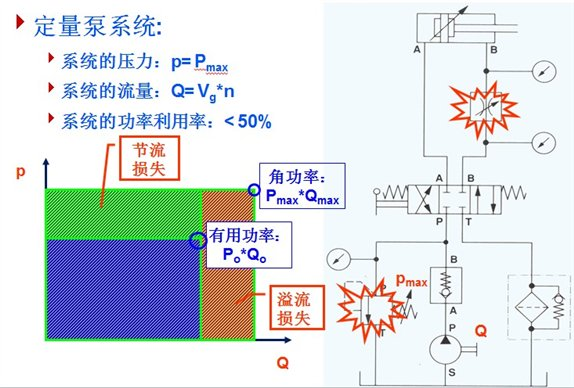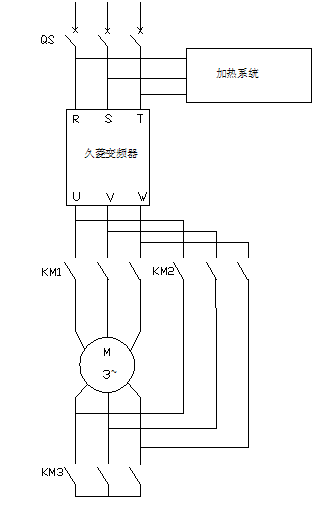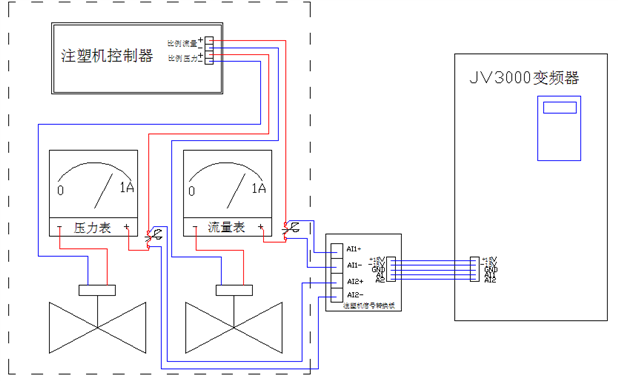P0.01=1

P0.07=1

P0.08=2

P0.10=2

P0.11=1S

P0.12=1S

P0.14=4

P5.14=7（实际测定）

P5.15=50%（根据生产效率可调整）

P5.19=7（实际测定）

P5.20=50%（根据生产效率可调整）